# AP Statistics : How to find z-scores for a data set

## Example Questions

← Previous 1

### Example Question #1 : How To Find Z Scores For A Data Set

This past week, the temperature has fluctuated quite a bit. From Monday to Sunday, temperatures in Fahrenheit have been the following: 62, 68, 52, 40, 78, 72, 60. The standard deviation is given as 13 and does not need to be calculated. Convert these daily temperatures into z-scores and give the days that are at least one standard deviation away from the mean.

Thursday and Friday

Wednesday

Thursday

Thursday and Wednesday

Friday

Thursday and Friday

Explanation:

To convert the temperatures into the z-scores, subtract the mean temperature (61.7) from the daily temperatures and divide this by the given standard deviation (13). The daily z-scores are .02, .53, -.81, -1.8, 1.4, .86, and -.14. Recall that z-scores tell you how many standard deviations away from the mean a given observation is.

Only Thursday and Friday are greater than one.

### Example Question #1 : How To Find Z Scores For A Data Set

The average score on the statistics final exam was 85 and the standard deviation was. Chris scored a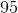. Chris scored higher than what percent of his class?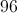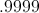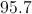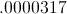Explanation:

The first step in this problem is calculating the z-score.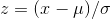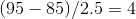The next step is to look up 4 in the z-table. The value from the table is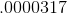.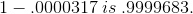### Example Question #1 : How To Find Z Scores For A Data Set

What is the-score for a value of 115 when the mean of the population is 103 and the standard deviation is 8?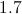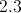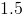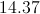Explanation:-score indicates whether a particular value is typical for a population or data set.  The closer the-score is to 0, the closer the value is to the mean of the population and the more typical it is.  The-score is calculated by subtracting the mean of a population from the particular value in question, then dividing the result by the population's standard deviation.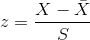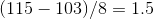### Example Question #1 : How To Find Z Scores For A Data Set

A population of values has a mean of 43 and a standard deviation of 12.  One of the values in the population is 49.  What is the Z-score for that value?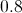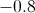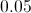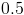Explanation:

A Z-score indicates whether a particular value is typical for a population or data set.  The closer the Z-score is to 0, the closer the value is to the mean of the population and the more typical it is.  The Z-score is calculated by subtracting the mean of a population from the particular value in question, then dividing the result by the population's standard deviation.

### Example Question #1 : How To Find Z Scores For A Data Set

The z-score is also known as the standard score.

The average temperature for all the days preceding the last for the month of February was 55 degrees Farhenheit, with a standard deviation of 5 degrees.

On the last day, the temperature was 73 degrees F. What is the z-score for the temperature on the last day?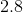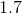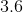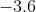Explanation:

To find the z-score, follow the formula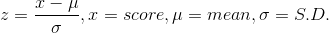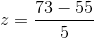or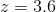### Example Question #1 : How To Find Z Scores For A Data Set

A population has a standard deviation of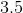and a mean of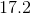. One of the values in the population is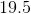.  What is the z score for that value?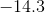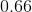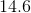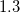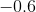Explanation:

A z score is unique to each value within a population.

To find a z score, subtract the mean of a population from the particular value in question, then divide the result by the population's standard deviation.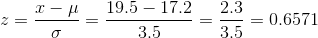### Example Question #1 : How To Find Z Scores For A Data Set

Natalie took her university placement examinations in Spanish and math. In Spanish, she scored 82; in math, she scored 86. The results of the Spanish exam had a mean of 72 and a standard deviation of 8. The results of the math exam had a mean of 68 and a standard deviation of 12. On which exam did Natalie do better, compared with the rest of her peers taking these placement exams?

She did equally well on both exams

The Math exam

The Spanish exam

None of the other answers, as z-scores cannot be calculated for this question

The Math exam

Explanation:

We need to calculate Natalie's z-scores for both her Spanish and math exams. Calculating z-scores is as follows: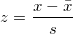Her z-score for the Spanish exam is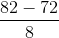, which equals 1.25, while her z-score for the math exam is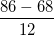, which equals 1.50. Since both z-scores are positive, Natalie did above average on both tests, but since her z-score for the math exam is higher than her z-score for the Spanish exam, Natalie did better on her math exam when compared to the rest of her peers taking the exam.

### Example Question #1 : Data Sets And Z Scores

The following data set represents Mr. Marigold's students' scores on the final. The standard deviation for this data set is 8.41. How many standard deviations are you away from the mean if you scored an 86? [find your z-score]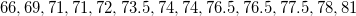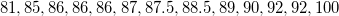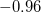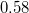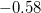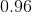Explanation:

To calculate the z-score, first we need to find the mean of the data set. By adding together and dividing by 26, we get 81.15.

To calculate your z-score and discover how close your score is to the mean in terms of standard deviations, use this formula: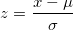where x is your data point, 86,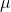is the mean, 81.15, and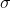is the standard deviation, which we are told is 8.41.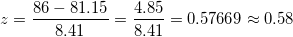### Example Question #1 : Data Sets And Z Scores

The following data set represents Mr. Marigold's students' scores on the final. The standard deviation for this data set is 8.41. How many standard deviations are you away from the mean if you got all the questions right? [find your z-score]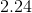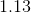Explanation:

To calculate the z-score, first we need to find the mean of the data set. By adding together and dividing by 26, we get 81.15.

To calculate your z-score and discover how close your score is to the mean in terms of standard deviations, use this formula:where x is your data point, 100,is the mean, 81.15, andis the standard deviation, which we are told is 8.41.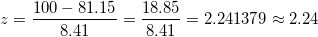### Example Question #1 : How To Find Z Scores For A Data Set

This year's harvest, the apples had a mean mass of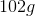with a standard deviation of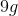. You pick an apple from the harvest and find its mass to be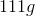.

What is the z-score of the apple you picked?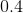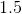Explanation:

To find the z-score, we follow the formula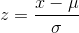whereis the given value,is the mean, andis the standard deviation.

For this problem we see that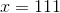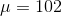and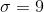Substituting for these values we see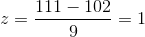← Previous 1

### All AP Statistics Resources